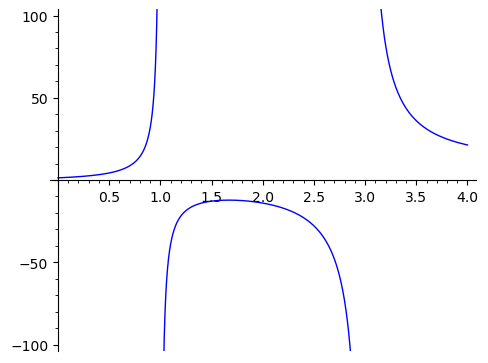# ymin and ymax not working

Running this code

f_x = (x**3 - x**2 + 3*x + 4) / (x**2 - 4*x + 3)
plot(f_x, (x, 0, 4), detect_poles=True, ymin=-100, ymax=100)


results in a graph with a max y of about 500000.

What am I doing wrong? The x-axis is limited, as I want, in the range 0 to 4.

edit retag close merge delete

Sort by » oldest newest most voted

Not sure what the problem might be with your installation.

It could help to know the operating system, the version of Sage, and the way it was it installed.

The output I get with Sage 9.5.beta4 built from source on macOS 10.14.6 is correct.

sage: f_x = (x**3 - x**2 + 3*x + 4) / (x**2 - 4*x + 3)
sage: plot(f_x, (x, 0, 4), detect_poles=True, ymin=-100, ymax=100)
Launched png viewer for Graphics object consisting of 3 graphics primitivesLikewise on SageCell, currently running Sage 9.4.

more

Does this work?

f_x = (x**3 - x**2 + 3*x + 4) / (x**2 - 4*x + 3)
_ = plot(f_x, (x, 0, 4), detect_poles=True)
_.show(ymin=-100, ymax=100)

more